Test: Fourier Series, Numerical Methods & Complex Variables- 1

# Test: Fourier Series, Numerical Methods & Complex Variables- 1 - Mechanical Engineering

Test Description

## 20 Questions MCQ Test GATE Mechanical (ME) 2024 Mock Test Series - Test: Fourier Series, Numerical Methods & Complex Variables- 1

Test: Fourier Series, Numerical Methods & Complex Variables- 1 for Mechanical Engineering 2023 is part of GATE Mechanical (ME) 2024 Mock Test Series preparation. The Test: Fourier Series, Numerical Methods & Complex Variables- 1 questions and answers have been prepared according to the Mechanical Engineering exam syllabus.The Test: Fourier Series, Numerical Methods & Complex Variables- 1 MCQs are made for Mechanical Engineering 2023 Exam. Find important definitions, questions, notes, meanings, examples, exercises, MCQs and online tests for Test: Fourier Series, Numerical Methods & Complex Variables- 1 below.
Solutions of Test: Fourier Series, Numerical Methods & Complex Variables- 1 questions in English are available as part of our GATE Mechanical (ME) 2024 Mock Test Series for Mechanical Engineering & Test: Fourier Series, Numerical Methods & Complex Variables- 1 solutions in Hindi for GATE Mechanical (ME) 2024 Mock Test Series course. Download more important topics, notes, lectures and mock test series for Mechanical Engineering Exam by signing up for free. Attempt Test: Fourier Series, Numerical Methods & Complex Variables- 1 | 20 questions in 60 minutes | Mock test for Mechanical Engineering preparation | Free important questions MCQ to study GATE Mechanical (ME) 2024 Mock Test Series for Mechanical Engineering Exam | Download free PDF with solutions
 1 Crore+ students have signed up on EduRev. Have you?
Test: Fourier Series, Numerical Methods & Complex Variables- 1 - Question 1

### The Fourier series of a real periodic function has only  P. cosine terms if it is even   Q. sine terms if it is even   R. cosine terms if it is odd   S. sine terms if it is odd  Which of the above statements are correct?

Detailed Solution for Test: Fourier Series, Numerical Methods & Complex Variables- 1 - Question 1

Because sine function is odd and cosine is even function.

Test: Fourier Series, Numerical Methods & Complex Variables- 1 - Question 2

### For the function e–x, the linear approximation around x = 2 is

Detailed Solution for Test: Fourier Series, Numerical Methods & Complex Variables- 1 - Question 2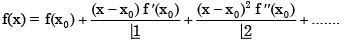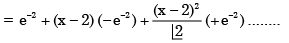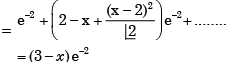(neglecting higher power of x)

Test: Fourier Series, Numerical Methods & Complex Variables- 1 - Question 3

### In the Taylor series expansion of exp(x) + sin(x) about the point x = π, the coefficient of (x – π)2 is

Detailed Solution for Test: Fourier Series, Numerical Methods & Complex Variables- 1 - Question 3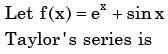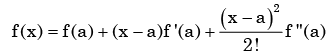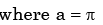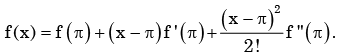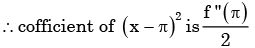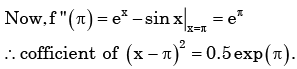Test: Fourier Series, Numerical Methods & Complex Variables- 1 - Question 4

The function x(t) is shown in the figure. Even and odd parts of a unit-step function u(t) are respectively,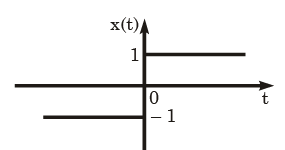Detailed Solution for Test: Fourier Series, Numerical Methods & Complex Variables- 1 - Question 4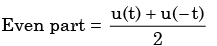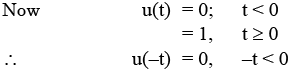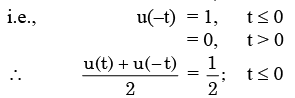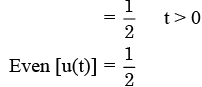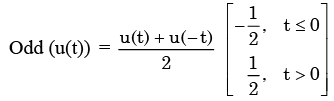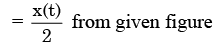Test: Fourier Series, Numerical Methods & Complex Variables- 1 - Question 5

In the Taylor series expansion of ex about x = 2, the coefficient of (x - 2)4 is

Detailed Solution for Test: Fourier Series, Numerical Methods & Complex Variables- 1 - Question 5

Taylor series expansion of f(x) about a is given by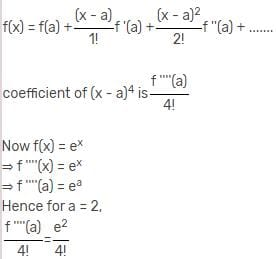Test: Fourier Series, Numerical Methods & Complex Variables- 1 - Question 6

The Fourier series expansion of a symmetric and even function, f(x) where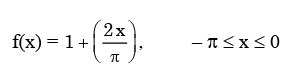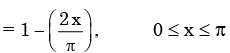Will be

Detailed Solution for Test: Fourier Series, Numerical Methods & Complex Variables- 1 - Question 6

f(x) is symmetric and even, it’s Fourier series contain only cosine term.  Now.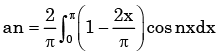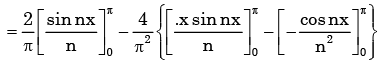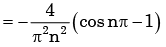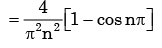Test: Fourier Series, Numerical Methods & Complex Variables- 1 - Question 7

The Fourier series expansion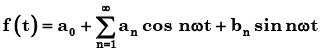of the periodic signal shown below will contain the following nonzero terms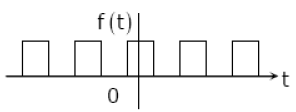Detailed Solution for Test: Fourier Series, Numerical Methods & Complex Variables- 1 - Question 7

from the figure, we can say that   f(t) is an symmetric and even function of t.  as cost is even function so choice (b) is correct.

Test: Fourier Series, Numerical Methods & Complex Variables- 1 - Question 8

The Fourier series for the function f(x)=sin2x is

Detailed Solution for Test: Fourier Series, Numerical Methods & Complex Variables- 1 - Question 8

Here f(x ) = sin2 x is even function, hence f( x ) has no sine term.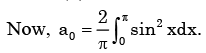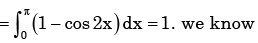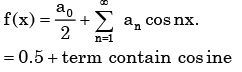Test: Fourier Series, Numerical Methods & Complex Variables- 1 - Question 9

X(t) is a real valued function of a real variable with period T. Its trigonometric Fourier Series expansion contains no terms of frequency ω = 2π (2k ) /T ; k = 1, 2,.... Also, no sine terms are present. Then x(t) satisfies the equation

Detailed Solution for Test: Fourier Series, Numerical Methods & Complex Variables- 1 - Question 9

No sine terms are present.

∴x(t ) is even function.

Test: Fourier Series, Numerical Methods & Complex Variables- 1 - Question 10

The residue of the function f(z)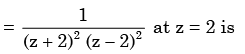Detailed Solution for Test: Fourier Series, Numerical Methods & Complex Variables- 1 - Question 10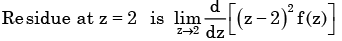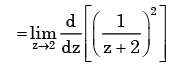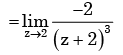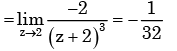Test: Fourier Series, Numerical Methods & Complex Variables- 1 - Question 11

The residues of a complex function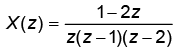​ at its poles are   ​

Detailed Solution for Test: Fourier Series, Numerical Methods & Complex Variables- 1 - Question 11

x(z) has simple poles at z = 0,1, 2.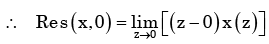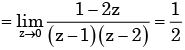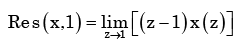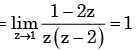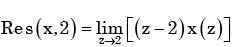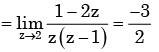Test: Fourier Series, Numerical Methods & Complex Variables- 1 - Question 12

The value of the contour integral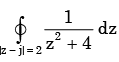in positive sense is

Detailed Solution for Test: Fourier Series, Numerical Methods & Complex Variables- 1 - Question 12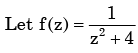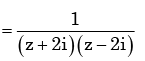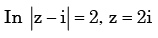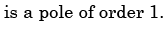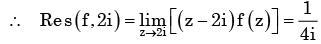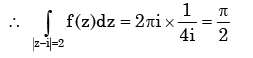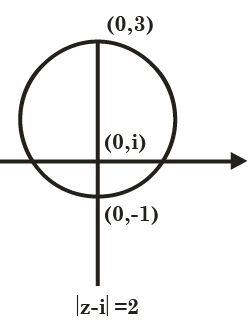Test: Fourier Series, Numerical Methods & Complex Variables- 1 - Question 13

The integral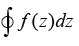evaluated around the unit circle on the complex plane for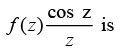Detailed Solution for Test: Fourier Series, Numerical Methods & Complex Variables- 1 - Question 13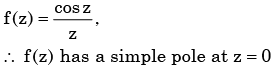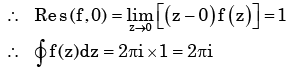Test: Fourier Series, Numerical Methods & Complex Variables- 1 - Question 14

An analytic function of a complex variable z = x + iy is expressed as f(z) = u (x, y) + i v(x, y) where i =√−1 . If u = xy, the expression for v should be

Detailed Solution for Test: Fourier Series, Numerical Methods & Complex Variables- 1 - Question 14

Here u and v are  analytic  as f(z) is analytic.

∴ u, v satisfy Cauchy-Riemann equation.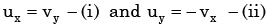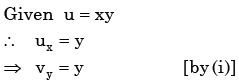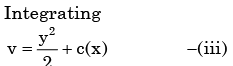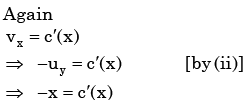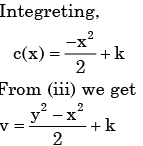Test: Fourier Series, Numerical Methods & Complex Variables- 1 - Question 15

The analytic function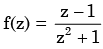has singularities at

Detailed Solution for Test: Fourier Series, Numerical Methods & Complex Variables- 1 - Question 15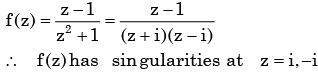Test: Fourier Series, Numerical Methods & Complex Variables- 1 - Question 16

The value of the integral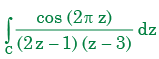(where C is a closed curve given by |z| = 1) is

Detailed Solution for Test: Fourier Series, Numerical Methods & Complex Variables- 1 - Question 16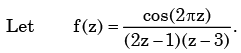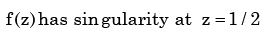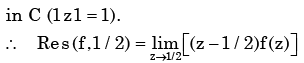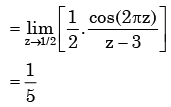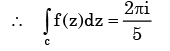Test: Fourier Series, Numerical Methods & Complex Variables- 1 - Question 17

Roots of the algebraic equation x3 +x2 +x+ 1 = 0 are

Detailed Solution for Test: Fourier Series, Numerical Methods & Complex Variables- 1 - Question 17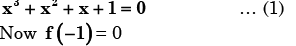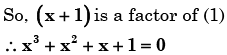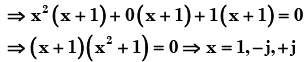Test: Fourier Series, Numerical Methods & Complex Variables- 1 - Question 18

The algebraic equation

F (s ) = s5 − 3s4+ 5s3− 7s2 + 4s + 20 is given F ( s ) = 0 has

Detailed Solution for Test: Fourier Series, Numerical Methods & Complex Variables- 1 - Question 18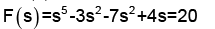we can solve it by making Routh Hurwitz array.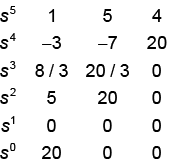We can replace 1st element of s1 by 10.

If we observe the 1st column, sign is changing two times.

So we have two poles on right half side of imaginary

Axis and 5s2+20=0

So, s =±2j and1 pole on left side of imaginary axis .

Test: Fourier Series, Numerical Methods & Complex Variables- 1 - Question 19

The value of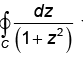Where C |z - 2i| = 1 is will be

Detailed Solution for Test: Fourier Series, Numerical Methods & Complex Variables- 1 - Question 19

Concept:

Cauchy's Integral Formula

If f(z) is analytic within and on a closed curve and if a is any point within C, then:

Analysis:

Given:

f(z)=∫1z2+4dz

|z - 2i| = 1

|x + iy - 2i| = 1

|x + i(y - 2)| = 1

Taking the magnitude of the above, we get:

x2 +(y - 2)2 = 1

This is the equation of a circle with:

Center = (0, 2)

Poles of f(z) = z2 + 4

z = (+2i, -2i)

2i lies Inside the circle

-2i lies outside the circle

∴ We can write:

Forpole(z=−2i)=∫1(z−2i)(z+2i)dz=0

Now, for pole z = 2i (lies inside the circle)

∫1(z+2i)(z−2i)dz=2πi[12i+2i]z=2i

Test: Fourier Series, Numerical Methods & Complex Variables- 1 - Question 20

Let z3 = z, where z is a complex number not equal to zero. Then z is a solution of

Detailed Solution for Test: Fourier Series, Numerical Methods & Complex Variables- 1 - Question 20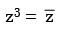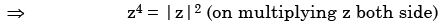Now by hit and trial method we see the solution being

Z4 = 1

## GATE Mechanical (ME) 2024 Mock Test Series

27 docs|243 tests
Information about Test: Fourier Series, Numerical Methods & Complex Variables- 1 Page
In this test you can find the Exam questions for Test: Fourier Series, Numerical Methods & Complex Variables- 1 solved & explained in the simplest way possible. Besides giving Questions and answers for Test: Fourier Series, Numerical Methods & Complex Variables- 1, EduRev gives you an ample number of Online tests for practice

## GATE Mechanical (ME) 2024 Mock Test Series

27 docs|243 tests